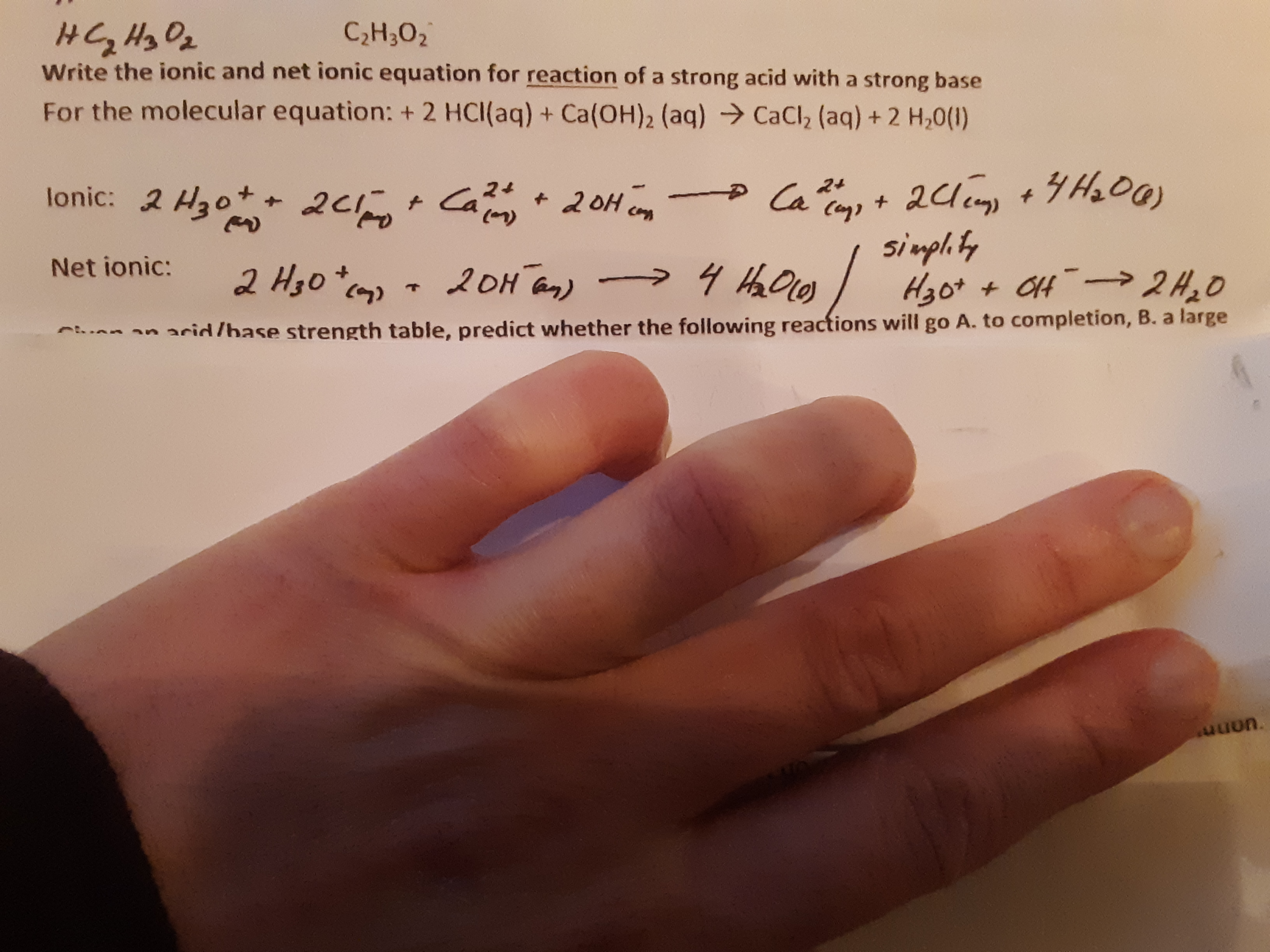# C2H302Write the ionic and net ionic equation for reaction of a strong acid with a strong baseFor the molecular equation: + 2 HCl(aq) + Ca(OH)2 (aq)CaCl2 (aq)+ 2 H,0()2d +H00)sinph tylonic: 2 H3o 2cCa24+ Ca24+20H2oH an)Net ionic:2 H3o+7an arid /hase strength table, predict whether the following reactions will go A. to completion, B. a largeסמn.

Question
2 views

In the equation (see pic below), how did we get hydronium ion in the beginning, instead of 2H+?help_outlineImage TranscriptioncloseC2H302 Write the ionic and net ionic equation for reaction of a strong acid with a strong base For the molecular equation: + 2 HCl(aq) + Ca(OH)2 (aq) CaCl2 (aq)+ 2 H,0() 2d +H00) sinph ty lonic: 2 H3o 2c Ca 24 + Ca 24 +20H 2oH an) Net ionic: 2 H3o + 7 an arid /hase strength table, predict whether the following reactions will go A. to completion, B. a large סמn. fullscreen
check_circle

star
star
star
star
star
1 Rating
Step 1

Net ionic Equation:

The net ionic equation shows only those chemical species that actually undergo chemical change.  The ions that do not change during the chemical reaction are called spectator i...

### Want to see the full answer?

See Solution

#### Want to see this answer and more?

Solutions are written by subject experts who are available 24/7. Questions are typically answered within 1 hour.*

See Solution
*Response times may vary by subject and question.
Tagged in

### Chemistry